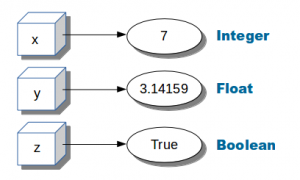# Python variablesVariables in Python (x,y,z). They can be used later in the program

Variables can hold numbers that you can use one or more times.

Numbers can be of one of these datatypes:

• integer (1,2,3,4)

• float (numbers behind the dot)

• boolean (True or False)

Related Course:
Complete Python Bootcamp: Go from zero to hero in Python 3## Numeric variables example

Example of numeric variables:

You can output them to the screen using the print() function.

Python supports arithmetic operations like addition (+), multiplication (*), division (/) and subtractions (-).

More mathematical operations

## User input

Python 3
Use the input() function to get text input, convert to a number using int() or float().

Python 2 (old version)
You can also ask the user for input using the raw_input function:

## 59 thoughts on “Python variables”

1.- May 29, 2016

” floats have numbers behind comma” Sir, I do not see any comma;perhaps you mean dot.
Please correct me if I am wrong.
Thank you.

1.- June 5, 2016

Yes, behind the dot.

2.- April 19, 2016

Correcting integer section replace from sys.maxint to sys.maxsize python 3.

3.- November 25, 2015

Do we have to use ” #!/usr/bin/env python ” always at the beginning?

1.- November 26, 2015

This depends on your computer. This is a line to indicate where Python is located. For example, on windows could be something like C:\Python\Python.exe

4.- November 18, 2015

num = input(‘Give me a number? ‘)
print(‘You said: ‘ + str(num)) pls explain the programme above.

1.- November 20, 2015

This program asks a number from the keyboard, this number is stored in the variable num. On the next line the variable num is shown on the screen.